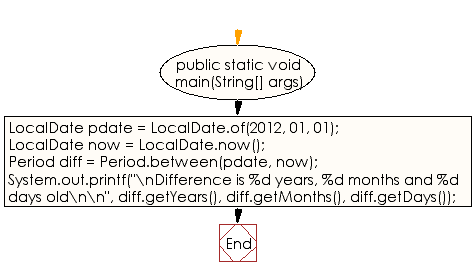﻿ Java exercises: Compute the difference between two dates(year, months, days) - w3resource# Java DateTime, Calendar Exercises: Compute the difference between two dates (year, months, days)

## Java DateTime, Calendar: Exercise-30 with Solution

Write a Java program to compute the difference between two dates (year, months, days).

Sample Solution:

Java Code:

``````import java.time.*;
import java.util.*;

public class Exercise1 {
public static void main(String[] args)
{
LocalDate pdate = LocalDate.of(2012, 01, 01);
LocalDate now = LocalDate.now();

Period diff = Period.between(pdate, now);

System.out.printf("\nDifference is %d years, %d months and %d days old\n\n",
diff.getYears(), diff.getMonths(), diff.getDays());
}
}
```
```

Sample Output:

```Difference is 5 years, 5 months and 20 days old
```

N.B.: The result may varry for your system date and time.

Flowchart:Java Code Editor:

Improve this sample solution and post your code through Disqus

What is the difficulty level of this exercise?

﻿

## Java: Tips of the Day

Directory Content:

Java allows you to get the names of all subdirectories and files in a folder as an array, which can then be sequentially viewed.

```import java.io.*;

public class ListContents {
public static void main(String[] args) {
File file = new File("//home//user//Documents/");
String[] files = file.list();

System.out.println("Listing contents of " + file.getPath());
for(int i=0 ; i < files.length ; i++)
{
System.out.println(files[i]);
}
}
}
```